Next: Angular distribution of radiation Up: Relativity and electromagnetism Previous: The Larmor formula

Radiation losses often determine the maximum achievable energy in a charged particle accelerator. Let us investigate radiation losses in various different types of accelerator device using the relativistic Larmor formula.

For a linear accelerator, the motion is one-dimensional. In this case, it is easily demonstrated that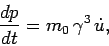(1650)

where use has been made of Eq. (1648), and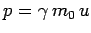is the particle momentum in the direction of acceleration (the-direction, say). Here,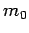is the particle rest mass. Thus, Eq. (1649) yields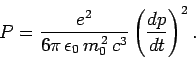(1651)

The rate of change of momentum is equal to the force exerted on the particle in the-direction, which, in turn, equals the change in the energy,, of the particle per unit distance. Consequently,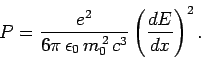(1652)

Thus, in a linear accelerator, the radiated power depends on the external force acting on the particle, and not on the actual energy or momentum of the particle. It is obvious, from the above formula, that light particles, such as electrons, are going to radiate a lot more than heavier particles, such as protons. The ratio of the power radiated to the power supplied by the external sources is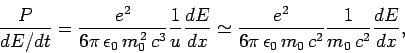(1653)

since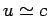for a highly relativistic particle. It is clear, from the above expression, that the radiation losses in an electron linear accelerator are negligible unless the gain in energy is of order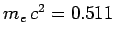MeV in a distance of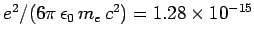meters. That is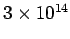MeV/meter. Typical energy gains are less that 10 MeV/meter. It follows, therefore, that radiation losses are completely negligible in linear accelerators, whether for electrons, or for other heavier particles.

The situation is quite different in circular accelerator devices, such as the synchrotron and the betatron. In such machines, the momentum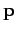changes rapidly in direction as the particle rotates, but the change in energy per revolution is small. Furthermore, the direction of acceleration is always perpendicular to the direction of motion. It follows from Eq. (1649) that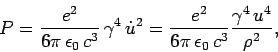(1654)

whereis the orbit radius. Here, use has been made of the standard result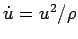for circular motion. The radiative energy loss per revolution is given by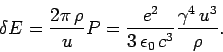(1655)

For highly relativistic () electrons, this expression yields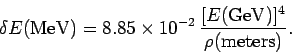(1656)

In the first electron synchrotrons,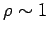meter,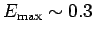GeV. Hence,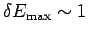keV per revolution. This was less than, but not negligible compared to, the energy gain of a few keV per turn. For modern electron synchrotrons, the limitation on the available radio-frequency power needed to overcome radiation losses becomes a major consideration, as is clear from the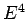dependence of the radiated power per turn.Next: Angular distribution of radiation Up: Relativity and electromagnetism Previous: The Larmor formula
Richard Fitzpatrick 2006-02-02